# Mms.php

 Mms.php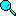Search

Author Message
Anonymous

 Posted on Tuesday, July 13, 2004 - 02:34 pm:

Hello
I found a code here to send mms in php but am null in php i don't understand it
someone can help and translate it in vb6.
here is the code

<?

{
\$dc = 0;
\$bo ="-----------------------------305242850528394";

\$fp = fsockopen(\$host, \$port, \$errno, \$errstr);
if (!\$fp) {
echo "errno: \$errno \n";
echo "errstr: \$errstr\n";
return \$result;
}

fputs(\$fp, "POST / HTTP/1.1\r\n");
echo "auth: \$auth\n";
\$auth = base64_encode(\$auth);
echo "auth: \$auth\n";
fwrite(\$fp, "Authorization: Basic " . \$auth . "\r\n");
}
fputs(\$fp, "User-Agent: NowSMS PHP Script\r\n");
fputs(\$fp, "Accept: */*\r\n");
fputs(\$fp, "Content-type: multipart/form-data; boundary=\$bo\r\n");

foreach(\$data_to_send as \$key=>\$val) {
\$ds =sprintf("%s\r\nContent-Disposition: form-data; name=\"%s\"\r\n%s\r\n",\$bo,\$key,\$val);
\$dc += strlen(\$ds);

}
\$dc += strlen(\$bo)+3;
fputs(\$fp, "Content-length: \$dc\r\n");
fputs(\$fp, "\r\n");
fputs(\$fp, "This is a MIME message\r\n\r\n");

foreach(\$data_to_send as \$key=>\$val) {
\$ds =sprintf("%s\r\nContent-Disposition: form-data; name=\"%s\"\r\n%s\r\n",\$bo,\$key,\$val);
fputs(\$fp, \$ds );
}
\$ds = \$bo."--\r\n" ;
fputs(\$fp, \$ds);

\$res = "";

while(!feof(\$fp)) {
}
fclose(\$fp);

return \$res;
}

\$file1="C:\TEMP\logo.gif";
\$contenttype1 = "image/gif";

\$fa = @file(\$file1);
\$xf ="Content-Type: ".\$contenttype1."\r\n\r\n".implode("",\$fa);
\$data["MMSFile\"; filename=\"\$file1"] = \$xf;

\$file2="C:\TEMP\test.smil";
\$contenttype2 = "application/smil";

\$fa = @file(\$file2);
\$xf="Content-Type: ".\$contenttype2."\r\n\r\n".implode("",\$fa);
\$data["MMSFile\"; filename=\"\$file2"] = \$xf;

/* Repeat this sequence for additional parts
\$file3="C:\TEMP\?????.???";
\$contenttype3 = "?????";

\$fa = @file(\$file3);
\$xf="Content-Type: ".\$contenttype3."\r\n\r\n".implode("",\$fa);
\$data["MMSFile\"; filename=\"\$file3"] = \$xf;
*/

/* A quirk of this script is that you must start all non-file parameters with \r\n */
\$data["MMSFrom"] = "\r\n" . "sender@domain.com";
\$data["PhoneNumber"] = "\r\n" . "+44999999999";
\$data["MMSSubject"] = "\r\n" . "Subject of message" ;

/* The MMSText field is optional */
\$data["MMSText" ] = "\r\n" . "Hello!" ;

\$x = PostToHost("127.0.0.1", 81, "test", "test", \$data);
echo \$x;

thank for help

Bryce Norwood - NowSMS Support

Post Number: 3053
Registered: 10-2002
 Posted on Wednesday, July 14, 2004 - 05:44 pm:

Unfortunately, I'm about as familiar with VB6 as you are with PHP.

Rather than translating the above code (which might be a bit of a challenge), it might be better to look at what is desired as the end result of the above code ... as there may be easier ways to accomplish the end result using VB.

Basically, what the above script is doing is submitting a web form which includes an HTTP file upload. (MIME type multipart/form-data) So maybe you can find some VB code that can do a form submission for an HTTP file upload?

We've got an example of what this format looks like at the following link: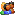Login / Register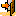Logout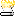Last 30 Days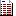Topics